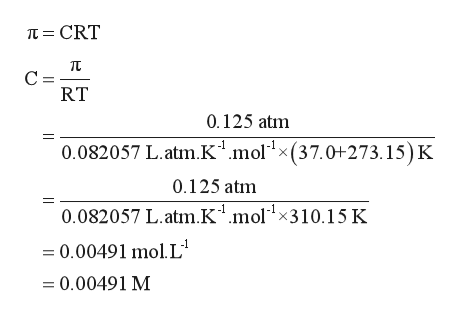# When 2.63 g of a polypeptide is dissolved in 101 mL of water, the resulting solution is found to have an osmotic pressure of 0.125 atm at 37.0 °C. What is the molar mass of the polypeptide?

Question
19 views

When 2.63 g of a polypeptide is dissolved in 101 mL of water, the resulting solution is found to have an osmotic pressure of 0.125 atm at 37.0 °C. What is the molar mass of the polypeptide?

check_circle

Step 1

Given information:

Mass of polypeptide = 2.63 g

Volume of water = 101 mL

Osmotic pressure = 0.125 atm

Temperature = 37.0 ℃

Step 2

Osmotic pressure (п) can be expressed in terms of concentration (C),gas constant (R), and temperature (T). The mathematical expression for osmotic pressure is represented as follows:

Step 3

Plugging the value of osmotic pressure, gas constant, and t...help_outlineImage Transcriptioncloset CRT TC C = RT 0.125 atm 0.082057 L. atm.K1.molx(37.0+273.15) K 0.125 atm 0.082057 L.atm.K1.molx310.15 K =0.00491 mol. L = 0.00491 M fullscreen

### Want to see the full answer?

See Solution

#### Want to see this answer and more?

Solutions are written by subject experts who are available 24/7. Questions are typically answered within 1 hour.*

See Solution
*Response times may vary by subject and question.
Tagged in

### Chemistry